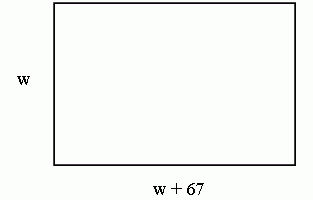SEARCH HOMEMath Central Quandaries & QueriesQuestion from Bobbi, a student: The Length of a football field is 67 yards greater than the width. The perimeter of the field is 346 yards. Find the length and width of the football fieldHi Bobbi,

One way to start is to let the width of the football field be w. Now, let's try and express what we know about the field in terms of w.

You told me that the length of the field is 67 yards more than the width. So, in terms of w, I can write: length = w + 67.

So now I have this picture of the field:Now, what else do you know about the field? You told me that the perimeter of the field is 346 yards. The perimeter is distance around the entire field. From the picture above, can you see how to write an expression in terms of w for the perimeter? Once you get this, you will have an equation that will let you solve for w.

Hope this helps,
HaleyMath Central is supported by the University of Regina and The Pacific Institute for the Mathematical Sciences.